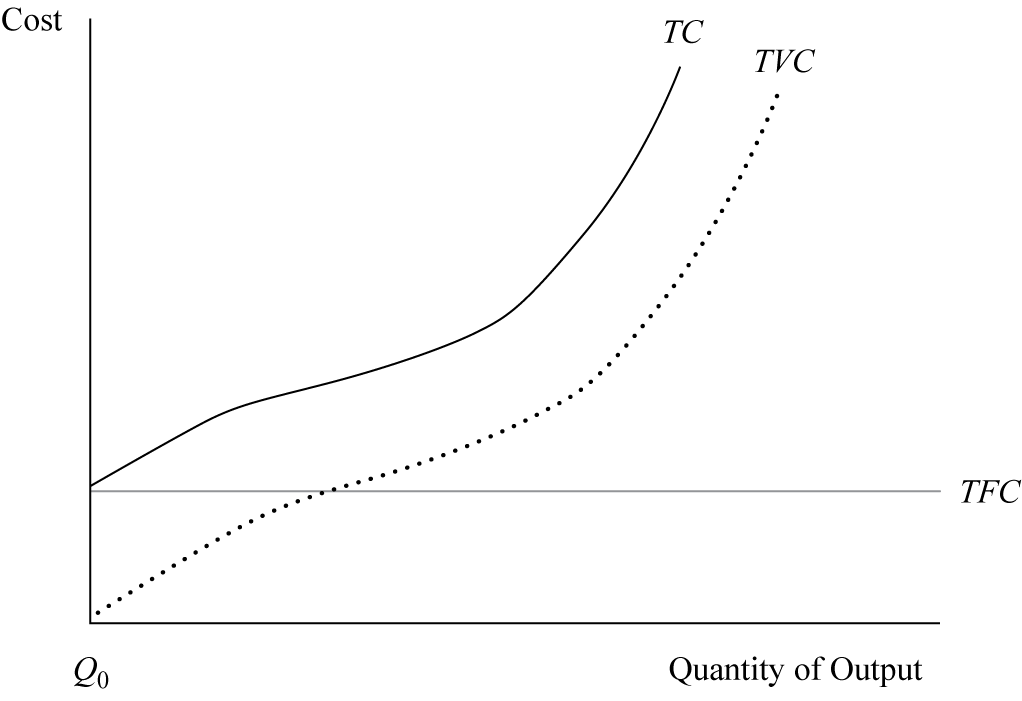Tags
#cfa-level-1 #economics #has-images #microeconomics #reading-15-demand-and-supply-analysis-the-firm #section-3-analysis-of-revenue-costs-and-profit #study-session-4Question
Explain, player.
It is the graphical relationships among total costs, total fixed cost, and total variable cost.

The curve for total costs is a parallel shift of the total variable cost curve and always lies above the total variable cost curve by the amount of total fixed cost.

At zero production, total costs are equal to total fixed cost because total variable cost at this output level is zero

Tags
#cfa-level-1 #economics #has-images #microeconomics #reading-15-demand-and-supply-analysis-the-firm #section-3-analysis-of-revenue-costs-and-profit #study-session-4Question
Explain, player.
?

Tags
#cfa-level-1 #economics #has-images #microeconomics #reading-15-demand-and-supply-analysis-the-firm #section-3-analysis-of-revenue-costs-and-profit #study-session-4Question
Explain, player.
It is the graphical relationships among total costs, total fixed cost, and total variable cost.

The curve for total costs is a parallel shift of the total variable cost curve and always lies above the total variable cost curve by the amount of total fixed cost.

At zero production, total costs are equal to total fixed cost because total variable cost at this output level is zero
If you want to change selection, open document below and click on "Move attachment"

Open it
Exhibit 10 shows the graphical relationships among total costs, total fixed cost, and total variable cost. The curve for total costs is a parallel shift of the total variable cost curve and always lies above the total variable cost curve by the amount of total fixed cost. At zero production, total costs are equal to total fixed cost because total variable cost at this output level is zero

#### Summary

status measured difficulty not learned 37% [default] 0

No repetitions